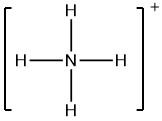QuestionAnswers

# The formal charge on nitrogen atom in ammonium ion is-(A) 3(B) 2(C) 1(D) 4

Hint: Try to identify the number of valence electrons present in each atom in the ammonium ${NH_4}^+$ ion. Recollect the Lewis dot structure of ammonium ion in order to find out the number of bonding and non-bonding electrons associated with each atom.

Complete solution:
Lewis structures or Lewis electron dot structures are simplified diagrams that represent arrangement of electrons in a molecule. Electrons are represented by using dots and lines are used for bonding between atoms.
Formal charge can be defined as the charge assigned to an atom in a molecule, making an assumption that electrons involved in chemical bonds have been shared equally between atoms, irrespective of their relative electronegativities.
In order to calculate formal charge, we need to assign electrons to individual atoms, present in a molecule.
Therefore, formal charge of each atom can be calculated by using the formula,
Formal charge = [(number of valence electrons in free atom) – (number of nonbonding electrons) + $\frac{1}{2}$ (number of bonding electrons)]
Now, let us consider the ammonium ion, whose Lewis structure can be drawn asWe can clearly see from the above Lewis structure that there are zero non-bonding electrons on nitrogen (N) atoms, and four bonds, i.e. eight bonding electrons. Also, the atomic number of N is 7, so the number of valence electrons will be 5.
Therefore, formal charge on N = [(5) – (0) – {( 1 /2 ) x 8}]
= 1

So, the correct option is C.

Note: If we will add all the formal charges of atoms present in a molecule, then we will get the total charge of that molecular ion. By definition, a molecule is neutral, i.e., possesses no charge. Formal charge is always calculated for an atom and not for molecules. The formal charge for a molecule is zero.